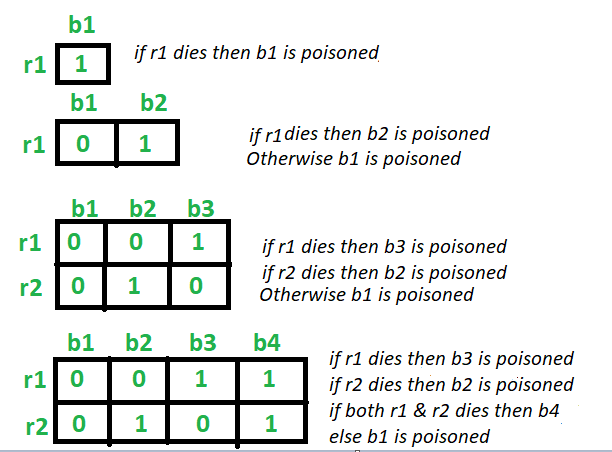# Rat and Poisoned bottle Problem

• Difficulty Level : Medium
• Last Updated : 06 Jun, 2021

Given N number of bottles in which one bottle is poisoned. So the task is to find out minimum number of rats required to identify the poisoned bottle. A rat can drink any number of bottles at a time.

Examples:

```Input: N = 4
Output: 2

Input: N = 100
Output: 7```

Approach:
Let’s start from the base case.

• For 2 bottles: Taking one rat (R1). If the rat R1 drinks the bottle 1 and dies, then bottle 1 is poisonous. Else the bottle 2 is poisonous. Hence 1 rat is enough to identify
• For 3 bottles: Taking two rats (R1) and (R2). If the rat R1 drinks the bottle 1 and bottle 3 and dies, then bottle 1 or bottle 3 is poisonous. So the rat R2 drinks the bottle 1 then. If it dies, then the bottle 1 is poisonous, Else the bottle 3 is poisonous.
Now if the rat R1 does not die after drinking from bottle 1 and bottle 3, then bottle 2 is poisonous.
Hence 2 rats are enough to identify.

• For 4 bottles: Taking two rats (R1) and (R2). If the rat R1 drinks the bottle 1 and bottle 3 and dies, then bottle 1 or bottle 3 is poisonous. So the rat R2 drinks the bottle 1 then. If it dies, then the bottle 1 is poisonous, Else the bottle 3 is poisonous.
Now if the rat R1 does not die after drinking from bottle 1 and bottle 3, then bottle 2 or bottle 4 is poisonous. So the rat R1 drinks the bottle 2 then. If it dies, then the bottle 2 is poisonous, Else the bottle 4 is poisonous.
Hence 2 rats are enough to identify.

• For N bottles:

Minimum number of rats required are = ceil(log2 N))Below is the implementation of the above approach:

## C++

 `// C++ program to implement``// the above approach` `#include ``using` `namespace` `std;` `// Function to find the minimum number of rats``int` `minRats(``int` `n)``{``    ``return` `ceil``(log2(n));``}` `// Driver Code``int` `main()``{``    ``// Number of bottles``    ``int` `n = 1025;` `    ``cout << ``"Minimum "` `<< minRats(n)``         ``<< ``" rat(s) are required"``         ``<< endl;` `    ``return` `0;``}`

## Java

 `// Java program to implement``// the above approach``class` `GFG``{``    ``public` `static` `double` `log2(``int` `x)``    ``{``        ``return` `(Math.log(x) / Math.log(``2``));``    ``}` `    ``// Function to find the minimum number of rats``    ``static` `int` `minRats(``int` `n)``    ``{``        ``return` `(``int``)(Math.floor(log2(n)) + ``1``);``    ``}``    ` `    ``// Driver Code``    ``public` `static` `void` `main (String[] args)``    ``{``        ``// Number of bottles``        ``int` `n = ``1025``;``    ` `        ``System.out.println(``"Minimum "` `+ minRats(n) +``                           ``" rat(s) are required"``);``    ``}   ``}` `// This code is contributed by AnkitRai01`

## Python3

 `# Python3 program to implement``# the above approach``import` `math` `# Function to find the``# minimum number of rats``def` `minRats(n):``    ` `    ``return` `math.ceil(math.log2(n));` `# Driver Code` `# Number of bottles``n ``=` `1025``;``print``(``"Minimum "``, end ``=` `"")``print``(minRats(n), end ``=` `" "``)``print``(``"rat(s) are required"``)` `# This code is contributed``# by divyamohan123`

## C#

 `// C# program to implement``// the above approach``using` `System;` `class` `GFG``{``    ``public` `static` `double` `log2(``int` `x)``    ``{``        ``return` `(Math.Log(x) / Math.Log(2));``    ``}` `    ``// Function to find the minimum number of rats``    ``static` `int` `minRats(``int` `n)``    ``{``        ``return` `(``int``)(Math.Floor(log2(n)) + 1);``    ``}``    ` `    ``// Driver Code``    ``public` `static` `void` `Main (String[] args)``    ``{``        ``// Number of bottles``        ``int` `n = 1025;``    ` `        ``Console.WriteLine(``"Minimum "` `+ minRats(n) +``                          ``" rat(s) are required"``);``    ``}``}` `// This code is contributed by 29AjayKumar`

## Javascript

 ``

Output:

`Minimum 11 rat(s) are required`

My Personal Notes arrow_drop_up